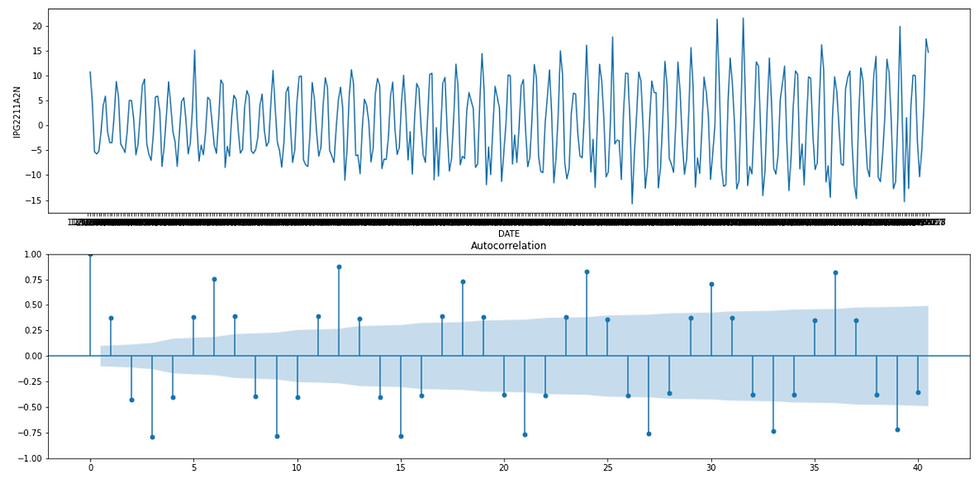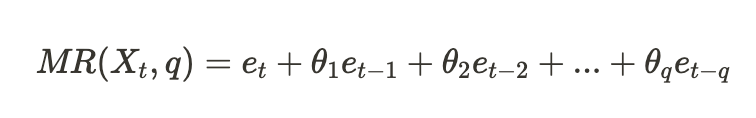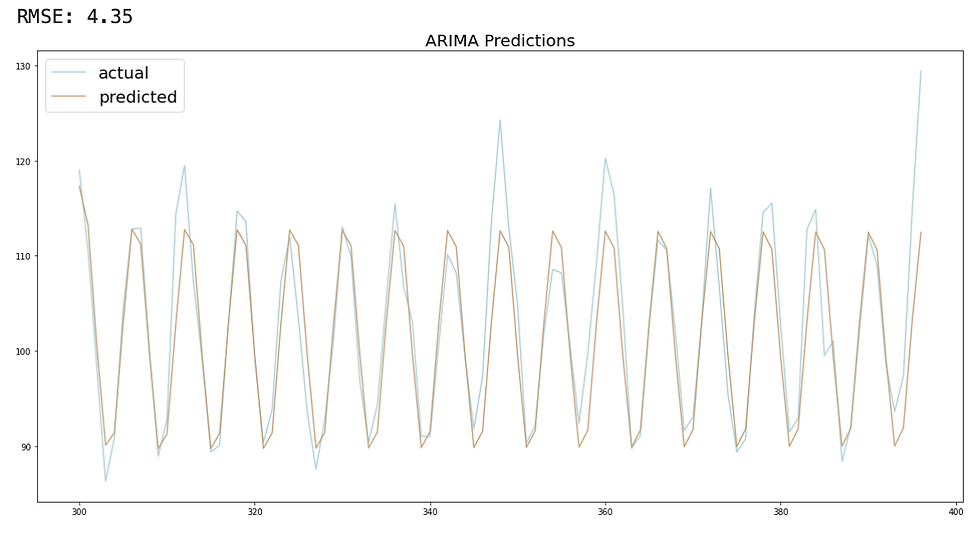top of pageSearch

# Time Series Analysis - ARMA, ARIMA, SARIMA

Updated: Dec 28, 2022

## What’s Their Difference and How to Use Them?## What is Time Series?

Time series is a unique type of problem in machine learning where the time component plays a critical role in the model predictions. As observations are dependent on adjacent observations, this violates the assumption that observations are independent to each other followed by most conventional machine learning models. Common use cases of time series analysis are forecasting future numeric values, e.g. stock pricing, revenue, temperature, which falls under the category of regression models. However, time series models can also be applied in classification problems, for instance, pattern recognition in brain wave monitoring, or failure identification in the production process are common applications of time series classifiers.

In this article, we will mainly focus on three time series model - ARMA, ARIMA, and SARIMA for regression problems where we forecast numeric values. Time series regression differentiates from other regression models, because of its assumption that data correlated over time and the outcomes from previous periods can be used for predicting the outcomes in the subsequent periods.

## How to Describe Time Series Data?

Firstly we can describe the time series data through a line chart visualization using sns.lineplot. As shown in the image below, the visualization of "Electric Production" time series data depicts an upward trend with some repetitive patterns.

```df = pd.read_csv("../input/time-series-datasets/Electric_Production.csv")
sns.lineplot(data=df,x='DATE', y='IPG2211A2N')```Time Series Visualization

To explain the characteristics of the time series data better, we can break it down into three components:

• trend - T(t): a long-term upward or downward change in the average value.

• seasonality - S(t): a periodic change to the value that follows an identifiable pattern.

• residual - R(t): random fluctuations in the time series data that does not follow any patterns.

They can be combined typically through addition or multiplication:

• Additive Time Series: O(t) = T(t) + S(t) + R(t)

• Multiplicative Time Series: O(t) = T(t) * S(t) * R(t)

In Python, we decompose these three components from time series data through seasonal_decompose, and decomposition.plot() gives us the visual breakdown of trend, seasonality and residual. In this code snippet, we specify the model to be additive and period = 12 to show the seasonal patterns.

```from statsmodels.tsa.seasonal import seasonal_decompose
decomposition = seasonal_decompose(x=df['IPG2211A2N'], model='additive', period = 12)
decomposition.plot()```Time Series Decomposition

## Stationarity

Time series data can be classified into stationary and non-stationary. Stationarity is an important property, as some models work well when the data is stationary. However, time series data often possesses the non-stationary property. Therefore, we need to understand how to identify non-stationary time series and how to transform it through various techniques, e.g. differencing.

Stationary data is defined as not depending on the time component and possesses the following characteristics, constant mean, constant variance overtime and constant autocorrelation structure (i.e., the pattern of autocorrelation does not change over time), without periodic or seasonal component.

### Techniques to Identify Stationarity

The most straightforward method would be examining the data visually. For example, the visualization above indicates that the time series follows an upward trend and its mean values increase over time, suggesting that the data is non-stationary. To quantify it stationarity, we can use following two methods.

Firstly, ADF (Augmented Dickey Fuller) test examines stationarity based on the null hypothesis that data is non-stationary and alternative hypothesis that data is stationary. If the p-value generated from the ADF test is smaller than 0.05, it provides stronger evidence to reject that data is non-stationary.

We can use adfuller from statsmodels.tsa.stattools module to perform the ADF test and generates the ADF value and p-value. In this example, p-value 0.29 is more than 0.05 thus this dataset is non-stationary.Secondly, ACF (Autocorrelation Function) summarizes the two-way correlation between the current observation against past observations. For example, when the lag=1 (x-axis), ACF value (y-axis) is roughly 0.85, meaning that the average correlation between all observations and their previous observation is 0.85. In the later section, we will also discuss using ACF to determine the moving average parameter.

The code snippet below generates ACF plots using sm.graphics.tsa.plot_acf, showing 40 lags.

```import statsmodels.api as sm
sns.lineplot(x=df['DATE'],  y=df['IPG2211A2N'], ax=subplot1)
sm.graphics.tsa.plot_acf(df['IPG2211A2N'], lags=40, ax=subplot2)
fig.show()```For non-stationary data, ACF drops to 0 relatively slowly, because non-stationary data may still appear highly correlated with previous observations, indicating that time component still plays an important role. The diagram above shows the ACF of the original time series data, which decreases slowly thus very likely to be non-stationary.

### Stationarity and Differencing

Differencing removes trend and seasonality by computing the differences between an observation and its subsequent observations, differencing can transform some non-stationary data to stationary.

1. remove trend

We use shift(1) to shift the original time series data (shown on the left) for one row down (shown on the right) and take the difference to remove the trend components. "dropna" is to remove the empty row when NaN is subtracted.

```# remove trend component
diff = df['IPG2211A2N'] - df['IPG2211A2N'].shift(1)
diff = diff.dropna(inplace=False)```We can plot the time series chart as well as the ACF plot after applying trend differencing. As shown below that the trend has been removed from the data and data appear to have constant mean. The next step is to address the seasonal component.

```# ACF after trend differencing
fig = plt.figure(figsize=(20, 10))
sns.lineplot(x=df['DATE'],  y=diff, ax=subplot1)
sm.graphics.tsa.plot_acf(diff, lags=40, ax=subplot2)
fig.show()```Trend Differencing

2. remove seasonality

From the ACF plot above, we can see that observations are more correlated when lag is 12, 24, 36 etc, thus it may follow a lag 12 seasonal pattern. Let us apply shift(12) to remove the seasonality and retest the stationarity using ADF - which has a p-value of around 2.31e-12.

```# remove seasonal component
diff = df['IPG2211A2N'] - df['IPG2211A2N'].shift(1)
seasonal_diff = diff - diff.shift(12)
seasonal_diff = seasonal_diff.dropna(inplace=False)```

After removing the seasonal pattern, the time series data below becomes more random and ACF value drops to a stable range quickly.Seasonal Differencing

## Models - ARMA, ARIMA, SARIMA

In this section, we will introduce three different models - ARMA, ARIMA and SARIMA for time series forecasting. Generally, the functionalities of these models can be summarized as follow:

• ARMA: Autoregressive + Moving Average

• ARIMA: Autoregressive + Moving Average + Trend Differencing

• SARIMA: Autoregressive + Moving Average + Trend Differencing + Seasonal Differencing

### ARMA - Baseline Model

ARMA stands for Autoregressive Moving Average. As the name suggests, it is a combination of two parts - Autoregressive and Moving Average.

Autoregressive Model - AR(p)

Autoregressive model makes predictions based on previously observed values, which can be expressed as AR(p) where p specifies the number of previous data points to look at. As stated below, where X represents observations from previous time points and φ represents the weights.For example, if p = 3, then the current time point is dependent on the values from previous three time points.

How to determine the p values?

PACF (Partial Autocorrelation Function) is typically used for determining p values. For a given observation in a time series Xt, it may be correlated with a lagged observation Xt-3 which is also impacted by its lagged values (e.g. Xt-2, Xt-1 ). PACF visualizes the direct contribution of the past observation to the current observations. For example, the PACF below when lag = 3 the PACF is roughly -0.60, which reflects the impact of lag 3 on the original data point, while the compound factor of lag 1 and lag 2 on lag 3 are not explained in the PACF value. The p values for the AR(p) model is then determined by when the PACF drops to below significant threshold (blue area) for the first time, i.e. p = 4 in this example below.PACF

Moving Average Model - MR(q)

Moving average model, MR(q) adjusts the model based on the average predictions errors from previous q observations, which can be stated as below, where e represents the error terms and θ represents the weights. q value determines the number of error terms to include in the moving average window.How to determine the q value?

ACF can be used for determining the q value. It is typically selected as the first lagged value of which the ACF drops to nearly 0 for the first time. For example, we would choose q=4 based on the ACF plot below.ACF

To build a ARMA model, we can use ARIMA function (which will be explained in the next section) in statsmodels.tsa.arima.model and specify the hyperparameter - order(p, d, q). When the d = 0, it operates as an ARMA model. Here we fit the ARIMA(p=3 and q=4) model to the time series data df“IPG2211A2N”.

```from statsmodels.tsa.arima.model import ARIMA
ARMA_model = ARIMA(df['IPG2211A2N'], order=(3, 0, 4)).fit()```

### Model Evaluation

Model evaluation becomes particularly important when choosing the appropriate hyperparameters for time series modeling. We are going to introduce three methods to evaluate time series models. To estimate model’s predictions on unobserved data, I used first 300 records in the original dataset for training and the remaining (from index 300 to 396) for testing.

```df_test = df[['DATE', 'IPG2211A2N']].loc[300:]
df = df[['DATE', 'IPG2211A2N']].loc[:299]```

1. Visualization

The first method is to plot the actual time series data and the predictions in the same chart and examine the model performance visually. This sample code firstly generates predictions from index 300 to 396 (same size as df_test) using the ARMA model, then visualizes the actual vs. predicted data. As shown in the chart below, since ARMA model fails to pick up the trend in the time series, the predictions drift away from actual values over time.

```# generate predictions
df_pred = ARMA_model.predict(start=300, end=396)
# plot actual vs. predicted
fig = plt.figure(figsize=(20, 10))
plt.title('ARMA Predictions', fontsize=20)
plt.plot(df_test['IPG2211A2N'], label='actual', color='#ABD1DC')
plt.plot(df_pred, label='predicted', color='#C6A477')
plt.legend(fontsize =20, loc='upper left')```2. Root Mean Squared Error (RMSE)

For time series regression, we can apply general regression model evaluation methods such as RMSE or MSE. For more details, please have a look at my article on "Top 4 Linear Regression Variations in Machine Learning".

Larger RMSE indicates more difference between actual and predicted values. We can use the code below to calculate the RMSE for the ARMA model - which is around 6.56.

```from sklearn.metrics import mean_squared_error
from math import sqrt
rmse = sqrt(mean_squared_error(df['IPG2211A2N'][1:], pred_df[1:]))
print("RMSE:", round(rmse,2))```

3. Akaike Information Critieria (AIC)

The third method is to use AIC, stated as AIC = 2k - 2ln(L), to interpret the model performance, which is calculated based on log likelihood (L) and number of parameters(k). We would like to optimize for a model to have less AIC, which means that:

• log likelihood needs to be high, so that models with high predictability would be preferred.

• the number of parameters is low, so that the model prediction is determined by fewer factors hence it is less likely to overfit and have a higher interpretability.

We can get the AIC value through summary() function, and the summary result below tells us that the ARMA model has AIC = 1527.26.

`ARMA_model.summary()`ARMA Model Summary

ARIMA stands for Autoregressive Integrated Moving Average, which extends from ARMA model and incorporates the integrated component (inverse of differencing).

ARIMA builds upon autoregressive model (AR) and moving average model (MA) by introducing degree of differencing components (specified as the parameter d) - ARIMA (p, d, q). This is to address when obvious trend has been observed in the time series data. As demonstrated in the ARMA example, the model didn’t manage to pick up the trend in the data which makes the predicted values drift away from the actual values.

In the “Stationarity and Differencing” section, we explained how differencing is applied to remove trend. Now let us explore how it makes the forecasts more accurate.

How to determine d value?

Since ARIMA incorporates differencing in its model building process, it does not strictly require the training data to be stationary. To ensure that ARIMA model works well, the appropriate degree of differencing should be selected, so that time series is transformed to stationary data after being de-trended.

We can use ADF test first to determine if the data is already stationary, if the data is stationary, no differencing is required hence d = 0. As mentioned previously, the ADF test before differencing gives us the p-value of 0.29.

After applying trend differencing (diff = df['IPG2211A2N'] - df['IPG2211A2N'].shift(1) ) and using ADF test , we found that p value is far below 0.05. Therefore, it indicates it is highly likely that transformed time series data is stationary.However, if the data is still non-stationary, a second degree of differencing might be necessary, which means applying another level of differencing to diff(e.g. diff2 = diff - diff.shift(1)).

To build the ARIMA model, we use the same function as mentioned in ARMA model and add the d parameter - in this example, d = 1.

```# ARIMA (p, d, q)
from statsmodels.tsa.arima.model import ARIMA
ARIMA_model = ARIMA(df['IPG2211A2N'], order=(3, 1, 4)).fit()
ARIMA_model.summary()```From the summary result, we can tell that the log likelihood increases and AIC decreases as compared to ARMA model, indicating that it has better performance.

The visualization also indicates that predicted trend is more aligned with the test data - with RMSE decreased to 4.35.SARIMA stands for Seasonal ARIMA which addresses the periodic pattern observed in the time series. Previously we have introduced how to use seasonal differencing to remove seasonal effects. SARIMA incorporates this functionality to predict seasonally changing time series and we can implement it using SARIMAX(p, d, q) x (P, D, Q, s). The first term (p, d, q) represents the order of the ARIMA model and (P, D, Q, s) represents the seasonal components. P, D, Q are the autoregressive, differencing and moving average terms of the seasonal order respectively. s is the number of observations in each period.

How to determine the s value?

ACF plot provides some evidence of the seasonality. As shown below, every 12 lags appears to have a higher correlation (as compared to 6 lags) to the original observation.We have also previously tested that after shifting the data with 12 lags, no seasonality has been observed in the visualization. Therefore, we specify s=12 in this example.

```#SARIMAX(p, d, q) x (P, D, Q, s)
SARIMA_model = sm.tsa.statespace.SARIMAX(df['IPG2211A2N'], order=(3, 1, 4),seasonal_order=(1, 1, 1, 12)).fit()
SARIMA_model.summary()```

From the summary result, we can see that AIC further decreases from 1528.48 for ARIMA to 1277.41 for SARIMA.The predictions now illustrates the seasonal pattern and the RMSE drops to 4.04## Take-Home Message

This introduction of time series models explains ARMA, ARIMA and SARIMA models in a progressive order.

• ARMA: Autoregressive + Moving Average

• ARIMA: Autoregressive + Moving Average + Trend Differencing

• SARIMA: Autoregressive + Moving Average + Trend Differencing + Seasonal Differencing

Furthermore, we explore concepts and techniques related to time series data, such as Stationarity, ADF test, ACF/PACF plot and AIC.